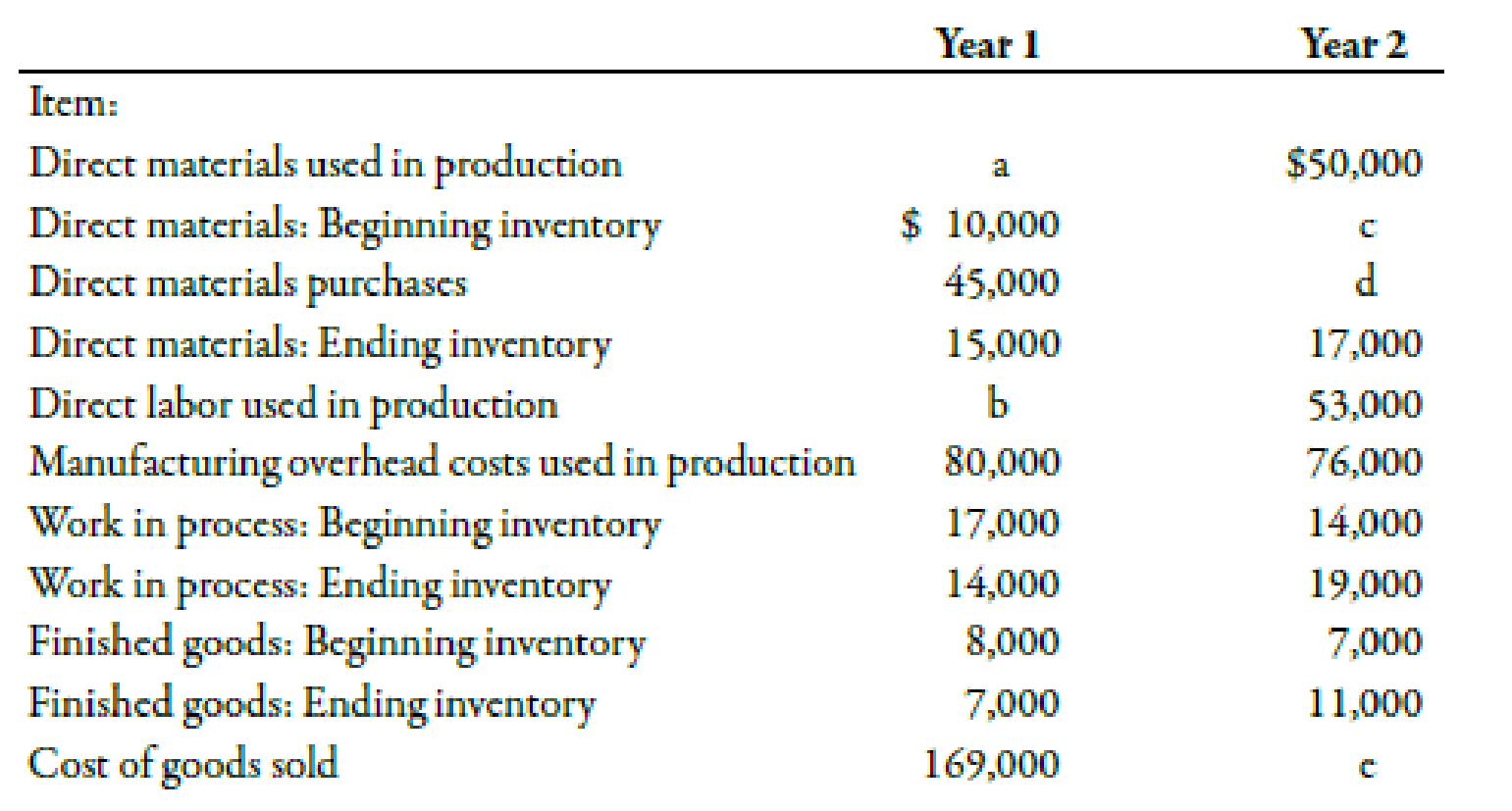Chapter 2, Problem 50E### Managerial Accounting: The Corners...

7th Edition
Maryanne M. Mowen + 2 others
ISBN: 9781337115773

#### Solutions

Chapter
Section### Managerial Accounting: The Corners...

7th Edition
Maryanne M. Mowen + 2 others
ISBN: 9781337115773
Textbook Problem
616 views

# Understanding the Relationship between Cost Flows, Inventories, and Cost of Goods SoldIvano Company has collected cost accounting information for the following subset of items for Years 1 and 2.Required:Calculate the values of the missing Items a through e.

To determine

Calculate the amounts of the missing items.

Explanation

Cost:

Cost can be defined as the cash and cash equivalent incurred against the products or its related services which will benefit the organization in the future. There are two types of costs that are direct and indirect costs.

Use the following formula to compute the amount of a (direct materials used in production for Year 1):

Direct materials used in production=(Begining inventory of materials+PurchasesEnding inventory of materials)

Substitute $10,000 for beginning inventory of materials,$45,000 for purchase and $15,000 for ending inventory of materials in the above formula. Direct materials used in production=$10,000+$45,000$15,000=$40,000 Therefore, the amount of direct materials used in production is$40,000.

Use the following formula to compute the amount of direct ‘b’ (direct labor used in production for Year 1):

Direct Labor=(Cost of goods soldBeginning finished goods+Ending finished goodsDirect materials used in productionManufacturing overheadBeginning WIP+Ending WIP)

Substitute $169,000 for the cost of goods sold,$8,000 for beginning finished goods, $7,000 for ending finished goods,$40,000 for direct materials used, $80,000 for manufacturing overhead,$17,000 for beginning WIP and $14,000 for ending WIP in the above formula. Direct Labor=($169,000$8,000+$7,000$40,000$80,000$17,000+$14,000)=$45,000 Therefore, the amount of direct labor used in production is$45,000

### Still sussing out bartleby?

Check out a sample textbook solution.

See a sample solution

#### The Solution to Your Study Problems

Bartleby provides explanations to thousands of textbook problems written by our experts, many with advanced degrees!

Get Started

#### Describe the three levels of management.

Foundations of Business (MindTap Course List)

#### In what ways is economics a science?

Essentials of Economics (MindTap Course List)

#### Should firms require higher rates of return on foreign projects than on identical projects located at home? Exp...

Fundamentals of Financial Management, Concise Edition (with Thomson ONE - Business School Edition, 1 term (6 months) Printed Access Card) (MindTap Course List)